Search
• danjhill95

# The Proofs are left in the Pudding for the Reader

I’m a big fan of mathematical proofs; one could argue that they are one of the few things that differentiate mathematics from other STEM subjects. It may not be the favourite topic of the average maths student, but there’s something special about being able to prove things in full generality. Also, creating your own proofs is a great exercise in logic, which is something I’d recommend for any maths students to at least try.

Here are some results that I've enjoyed finding proofs for in the past (which I’m leaving here for my own future reference). Some might be things that you were taught at school, and some others might surprise you:

1. Decimal expansion of one-seventh

First off, you may be aware that some fractions have a decimal expansion with infinitely repeating digits, such as 1/3 = 0.33333… One of the lesser known of these is 1/7, where its decimal has a repeating block of 6 digits “142857”. But how do we prove that this is true?

Let’s start by recognising that we can writeThen, we can do the same thing withFollowing this same approach, we see thatWhich, by dividing by the appropriate amount of 10’s, means thatApplying this recursively, we see that the 6-digit block “142857” just keeps on repeating infinitely.

2. A number is divisible by 3 if the sum of its digits is divisible by 3

This is a trick that many students learn to help with checking if a number is divisible by three but, other than being told this by our teachers, how do we know that this always works? Sure, we can check that it works for all numbers up to 200, say, but what if it stops working for all numbers larger than 1000? This is where a proof of full generality can really come into its own.

We first write the above statement in a bit more of a mathematical way. For some number A with N digits (so 134 would have N=3, 19928 would have N=5, etc.) we can write its decimal expansion aswhere each a, a, …, a[N-1] is the first, second, …, N’th digit of our number. So, for our previous examples,Then, our statement can be written as

A is divisible by 3 if and only if a + a + a + … + a[N-1] is divisible by 3.

To prove this, we recall that 10=9+1, and soWhich means that,Clearly, the last bracket is divisible by 3, since it is 9 times a collection of terms; hence, A is only divisible by 3 ifis divisible by 3. Playing the same trick, we find that A is only divisible by 3 ifis divisible by 3, and so on. Applying this N-1 times, we then arrive at the result.

3. The square root of 2 can't be written as a fraction of two whole numbers

Now this one, *slaps bonnet*, this is a classic. The fact that the square root of 2 cannot be written as a fraction of two whole numbers, which means that we call sqrt(2) irrational, is a staple of entry-level maths proofs in UK Universities. The thing that makes this problem so great is its accessibility. The proof itself only really uses properties of odd and even numbers.

To prove this, we’re going to use an idea known as proof by contradiction. What that means is, we are going to assume the statement is wrong and then follow the logic until we arrive at some contradiction. This would then mean that it is impossible for the statement to be wrong, and so it must be right.

Okay, so let’s assume that sqrt(2) can be written as a fraction of two whole numbers; that is, there exist some whole numbers p and q such thatNow, we can assume that p and q don’t have any common factors, since we could then simplify the fraction p/q by dividing through by this factor, i.e. if p=3*a, q=3*b for some whole numbers a and b, then we could write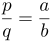The trick is to now square both sides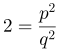And so, p^2 = 2*q^2. Therefore, p^2 is an even number, which also means that p is an even number. Then, we can writefor some whole number k, which means thatDividing by 2, we see thatwhich means that q^2, and thus q, is also even. But if q and p are both even, then they are both divisible by 2. This is a contradiction since p and q have no common factors. Hence, our assumption thatmust be wrong.

4. Pythagorean triples, quintuples, etc.

You may be aware of the identityfrom Pythagoras’s Theorem. Recently I saw the following result drifting around Math Twitter,Now, this kind of blew me away to start with, and it’s natural to ask `Is there a general version of this?’. In fact, there is, and so the statement I want to prove is the following:

For any whole number k>0, the equationhas one unique positive solution and one unique negative solution for a.

To prove this, I find it easier to first redefine the variable a; let’s introduce b=a+k such that the equation above becomesfor which we can rearrange into the following form: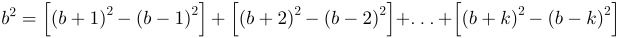We note thatand sowhich has two solutions: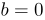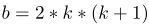Converting back to our variable a, we find thatare the two solutions. Hence, our equation has one unique positive solution and one unique negative solution. Since we’ve done this in full generality, we can now conclude thatand many more…

## 5. Checkerboard matrix negation is determinant-invariant

This final result is something I recently stumbled upon during some of my research, but it does have a bit more required knowledge regarding matrices. As such, I’m not going to go into the proof itself; instead, I’ll try to explain the result and then leave the proof as an exercise for any interested readers out there.

Firstly, it's important to know that a matrix is a collection of numbers in a row-column structure. For example,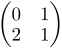is a 2x2 matrix (since there are 2 columns and 2 rows). For square matrices, where the number of rows and columns are the same, there is a measurement of the matrix known as the determinant that characterises its behaviour. Given a general 2x2 matrix A of the form,then the determinant is calculated asThe result I recently learned about is that, for any NxN matrix (where N is some positive whole number), negating every other element of the matrix, starting from the (row 1, column 2) element, leaves the determinant invariant. That is, given a matrix B of the formand let’s say that we define some new matrix C, of the form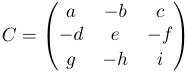Then det ( C ) = det ( B ). Note, this is true for any sized square matrix, which was something that astounded me.

I want to emphasise that, if you tried proving any of these results above as well as anything else, proofs are sometimes hard work. More than this, making a proof that is clear and easy to follow is even harder, and almost always requires multiple attempts. In fact, having noticed that the above result seemed to be true for the matrices I was working with, I managed to construct a 2-page proof by induction for the general case. Following this, I found an off-handed comment about this property in a paper where the author presented a two-line proof. So don’t be discouraged if you didn’t think up the most efficient proof for a particular result. The truth is, most of the proofs that you see are the results of multiple attempts, as well as multiple drafts to make them clear and concise.